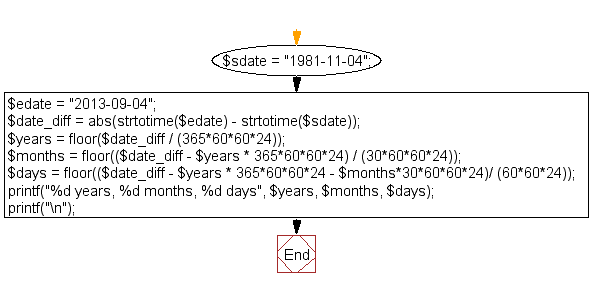﻿ PHP Date Exercise: Calculate the difference between two dates (in years, months, days) - w3resource# PHP Date Exercises : Calculate the difference between two dates (in years, months, days)

## PHP date: Exercise-4 with Solution

Write a PHP script to calculate the difference between two dates.

Sample dates : 1981-11-04, 2013-09-04

Sample Solution:

PHP Code:

``````<?php
\$sdate = "1981-11-04";
\$edate = "2013-09-04";

\$date_diff = abs(strtotime(\$edate) - strtotime(\$sdate));

\$years = floor(\$date_diff / (365*60*60*24));
\$months = floor((\$date_diff - \$years * 365*60*60*24) / (30*60*60*24));
\$days = floor((\$date_diff - \$years * 365*60*60*24 - \$months*30*60*60*24)/ (60*60*24));

printf("%d years, %d months, %d days", \$years, \$months, \$days);
printf("\n");
?>
```
```

Sample Output:

```31 years, 10 months, 12 days
```

Flowchart :PHP Code Editor:

Have another way to solve this solution? Contribute your code (and comments) through Disqus.

What is the difficulty level of this exercise?

﻿

## PHP: Tips of the Day

SQL injection that gets around mysql_real_escape_string()

Consider the following query:

```\$iId = mysql_real_escape_string("1 OR 1=1");
\$sSql = "SELECT * FROM table WHERE id = \$iId";
```

mysql_real_escape_string() will not protect you against this. The fact that you use single quotes (' ') around your variables inside your query is what protects you against this. The following is also an option:

```\$iId = (int)"1 OR 1=1";
\$sSql = "SELECT * FROM table WHERE id = \$iId";
```

Ref : https://bit.ly/32q3bJ7